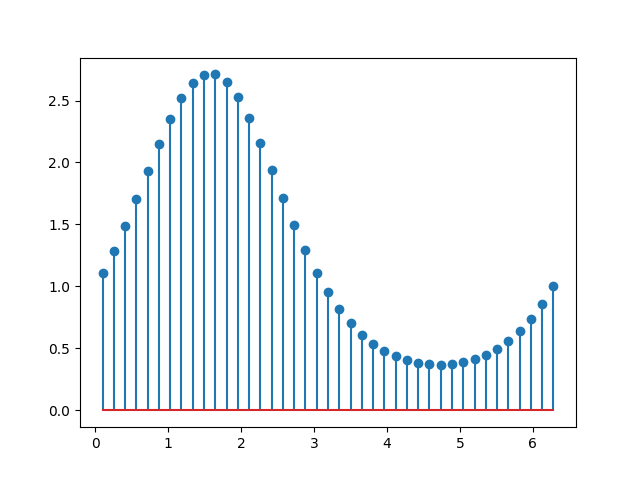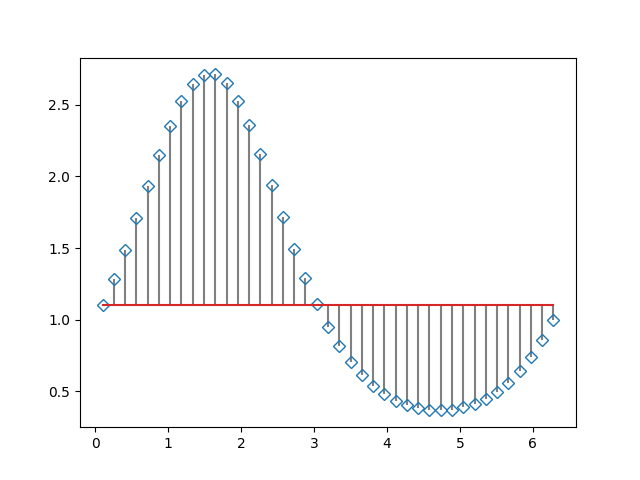# Stem Plot¶

stem plots vertical lines from a baseline to the y-coordinate and places a marker at the tip.

import matplotlib.pyplot as plt
import numpy as np

x = np.linspace(0.1, 2 * np.pi, 41)
y = np.exp(np.sin(x))

plt.stem(x, y)
plt.show()The position of the baseline can be adapted using bottom. The parameters linefmt, markerfmt, and basefmt control basic format properties of the plot. However, in contrast to plot not all properties are configurable via keyword arguments. For more advanced control adapt the line objects returned by pyplot.

markerline, stemlines, baseline = plt.stem(
x, y, linefmt='grey', markerfmt='D', bottom=1.1)
markerline.set_markerfacecolor('none')
plt.show()References

The use of the following functions, methods, classes and modules is shown in this example:

Keywords: matplotlib code example, codex, python plot, pyplot Gallery generated by Sphinx-Gallery